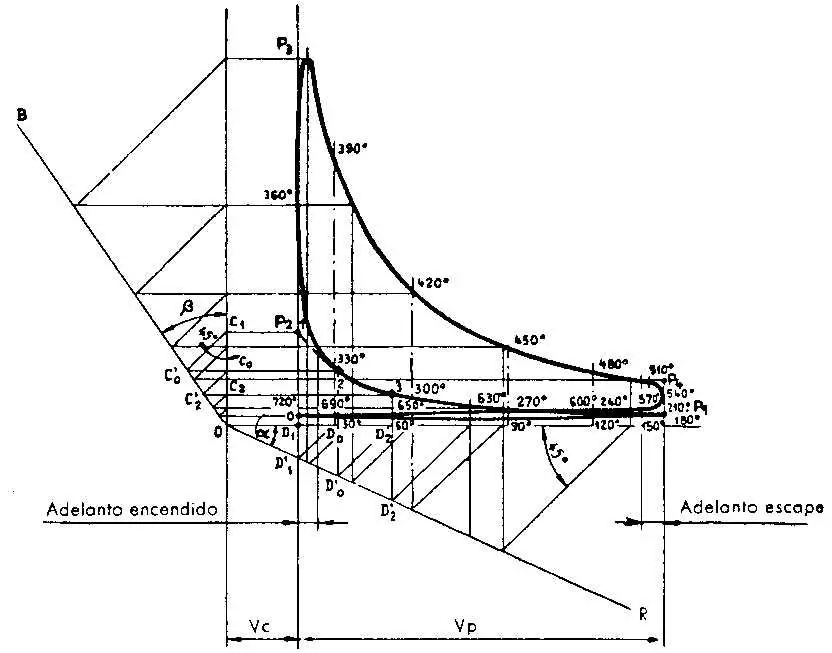# I cycle indicated provided

It is necessary to know the cycle, not only to check if the thermal engine operation is correct, but also to determine the value of the forces acting on the engine components. When in the design phase is to establish the dimensions of the engine components, proceed to the calculation of stresses based on an expected cycle. This similarity can be drawn by motor cycles parangonables with that study, but also obtained a simple and fast way, ore than with a sufficient approximation, using the following method is set forth by way of example and recommend as applicable to the case engine 4-stroke spark ignition.

Knowing the diameter, the race and the compression ratio p, calculate the volume of the compression chamber Vc, or that of the unit cylinder, Vp, whose Vc and Vc + Vp values we as a Cartesian diagram abscissa. Abscissa corresponding to said respective carry as ordinates pressures recorded at the end of each of the phases. In practice, for the Otto cycle values are fixed on a discrete approximation, as follows:

P1 = pressure at the end of aspiration = 0.9 to 1 Kg / cm2;
p2 = pressure at the end of compression p1 = p n;
p3 = maximum pressure at the end of combustion = 7 p - 2 ;
p4 = pressure cessation of expansion = p3 / p ne;

Wherein

N = exponent of the polytropic compression;
ne = exponent of the polytropic expansion.

Both polytropic are plotted as building Brauer. As an example to show how the compression curve is established, the OR line is drawn at an angle to either the x-axis, and then the line OB describing an angle b with the ordinate so that

Tg b = (1 + tg a) n -1

In which n = nc; or n ne.

Section 1 (p2) is projected on two Cartesian axes; then from the points C1 and D1 'two straight conn 45º opening axes are drawn, and from C'o and D'points or other two normal to said axis; the intersection of these gives us point 2 of the polytropic. Repeating the construction for point 2, point 3 is obtained, and so on. Similarly one proceeds to the expansion curve. Taking this when we explained about the difference between ideal cycle and the cycle indicated it will not be difficult to understand that appear rounded figure the various final strokes of the phases.In this illustration are indicated for each point corresponding angles of the crank.

For simplicity, the value of p1 is equal to 1 kg / cm2 for all cycles and all applications. P4 expression, indicated above, is also valid in all cases. For the Diesel cycle there is no empirical expressions which give values of p2 and p3 next to those calculated according to the rules and formulas of thermodynamics.

On engines for stationary and marine installations, given the limited range of variation of the compression ratio and the low priority that variation is about the high value of this ratio, generally not trace indicated the cycle provided for each motor but is part of a cycle type, standardized derivative evidence from existing engines with the indicator.

Scheduled cycle indicated thus obtained the stresses produced by the gas on the piston pin, multiplying the values of the surface pressure by the same piston deducted.

In the following table are given, for information, data media pressure and compression ratio couple both cycles and their various applications:

 Presiones: kg/cm2 Motores para aviación Motores para automoción Motores fijos y marinos Final de aspiración p1=1 Otto Diesel Otto Otto (a gas) Diesel Otto (a gas) Diesel Final de compresión p2 8-12 30-60 8.5-13 12-14 35-45 10-18 30-40 Màxima de combustión p3 30-60 55-100 38-52 40-50 55-70 20-40 60-70 Final de expansión p4 4.5-5.5 3.5 4-5 4-5 3 3-4 2.5-3.5 Relación de compresión 5.5-7.5 12-20 7-10 7-10 12-22 6-10 12-14
valoración: 3.1 - votos 7

Last review: February 1, 2016